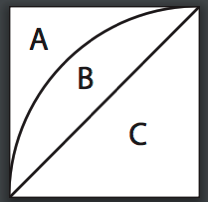Posted on

## Geometry Ch11 test #7b

Question from Anya
Course: Geometry
Chapter/Lesson/Problem11 test7b
Issue: I’m supposed to find Angle APM. To find the angle, I used tan APM=20/70, which gives 15.9 degrees. The solutions manual used sin APM=20/70, which gives 16.6 degrees. Sine is opposite/hypotenuse. However, it seems to me that the given measurements of the problem are opposite side (20) to the adjacent side (70). Which is correct? Thank you!
Yes, you are correct. That should be tangent as you stated.
tan(APM) = 20/70
Posted on

## Geometry Ch14 Test #4

Question: how do we work the problem on Chapter 14 test #4.

Since all 16 white regions are identical, we can find the area of one of them and multiply it by 16.

This figure represents one of the 16 small squares and one of the white regions.Since the diameter of the large circle in the figure shown on the test page is 4 units, the side of the square of one of the small squares is 1 unit.

We can find the area of region B by finding the area of regions B & C combined (a quarter of a circle) and subtracting the area of region C (a triangle):

> The area of B & C combined is1/4 (pi r squared) or 1/4 (pi 1 squared) = 1/4 pi

> The area of C is1/2 r squared or 1/2 1 squared = 1/2So B = (B + C) – C = 1/4 pi – 1/2 and the total area of the 16 white regions is16(1/4 pi – 1/2) = 4 pi – 8.

Posted on

## Geometry Midterm #74

Question from Kerry:

The answer key for the Geometry Midterm Review #74 says the answer is square root of 80, but shouldn’t it be the square root of 52?  It says (4-0) squared + (8-2)squared = 16+64, but it should be = 16+36, shouldn’t it?